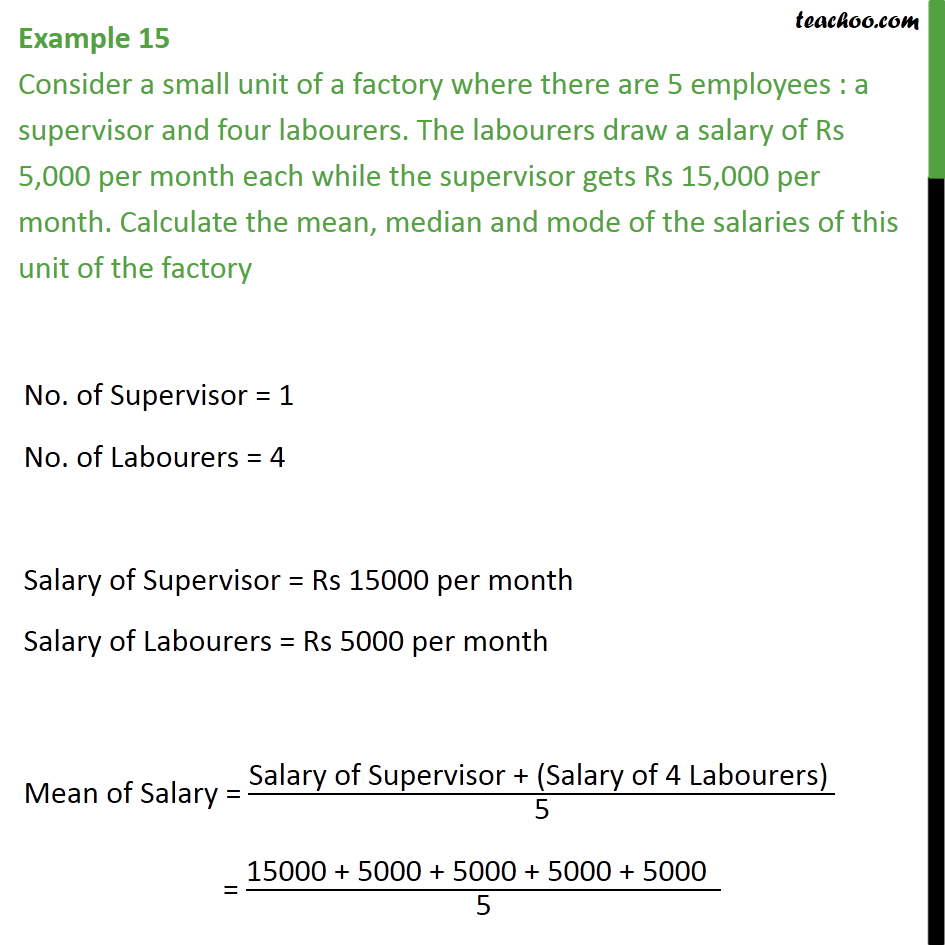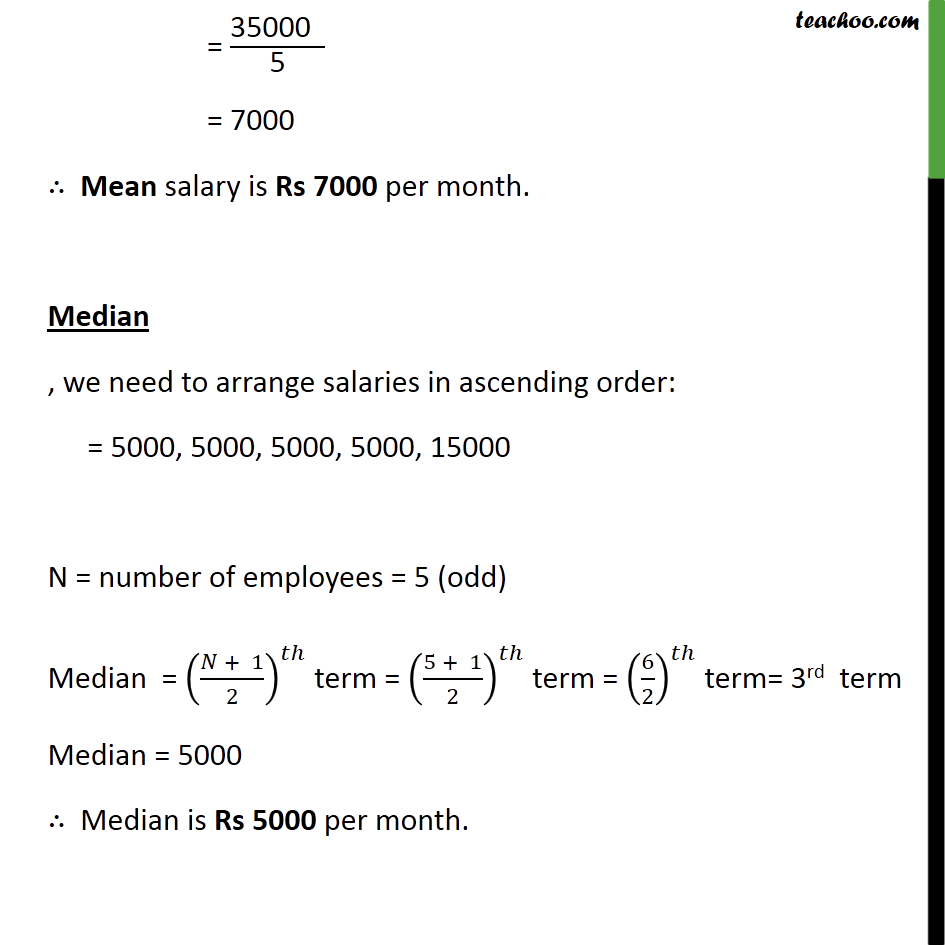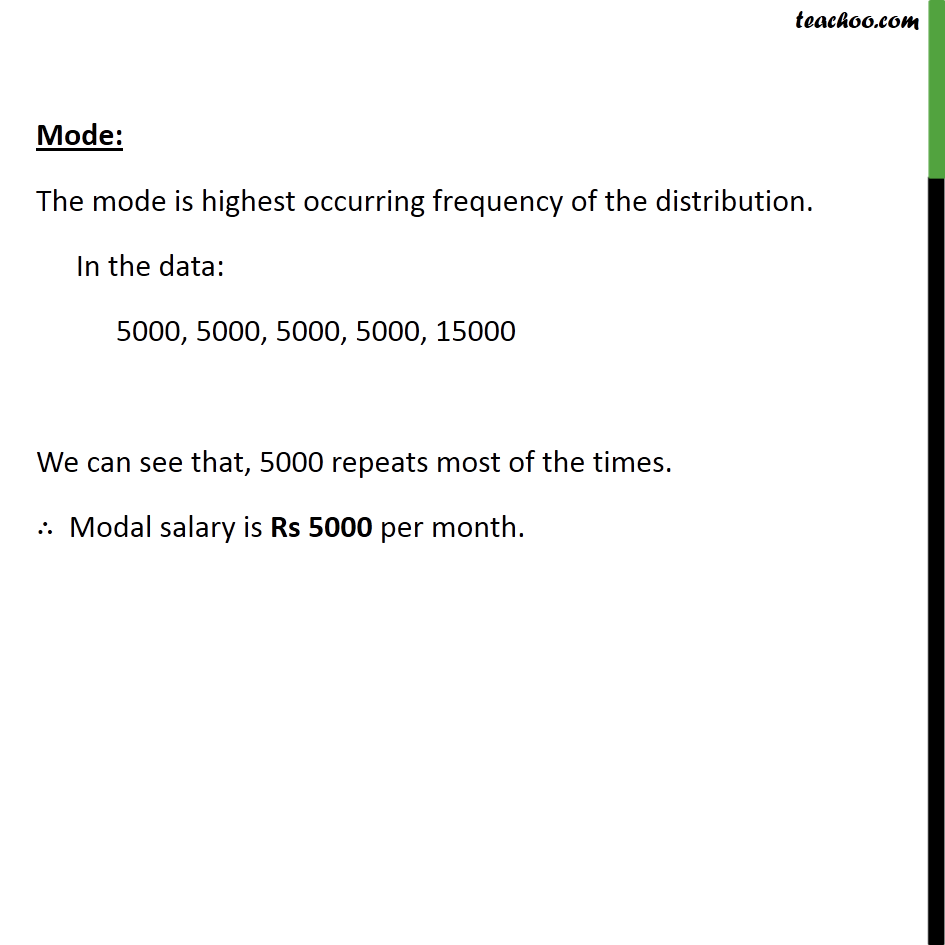Examples

Chapter 12 Class 9 Statistics
Serial order wiseLearn in your speed, with individual attention - Teachoo Maths 1-on-1 Class

### Transcript

Question 10 Consider a small unit of a factory where there are 5 employees : a supervisor and four labourers. The labourers draw a salary of Rs 5,000 per month each while the supervisor gets Rs 15,000 per month. Calculate the mean, median and mode of the salaries of this unit of the factory. = "35000 " /"5" = 7000 ∴ Mean salary is Rs 7000 per month. Median , we need to arrange salaries in ascending order: = "5000, 5000, 5000, 5000, 15000 " N = number of employees = 5 (odd) Median = ((𝑁 + 1)/2)^𝑡ℎ term = ((5 + 1)/2)^𝑡ℎ term = (6/2)^𝑡ℎ term= 3rd term Median = 5000 ∴ Median is Rs 5000 per month. Mode: The mode is highest occurring frequency of the distribution. In the data: 5000, 5000, 5000, 5000, 15000 We can see that, 5000 repeats most of the times. ∴ Modal salary is Rs 5000 per month.